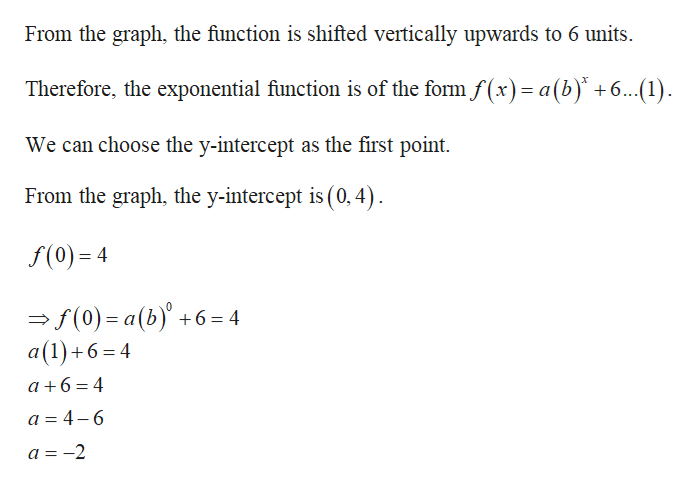# Find an exponential equation for the graph. y =

Question

Find an exponential equation for the graph.

y =

check_circleExpert Solution
Step 1

Formula:

Step 2

Diagram:

Step 3

Calculation:

...help_outlineImage TranscriptioncloseFrom the graph, the function is shifted vertically upwards to 6 units. Therefore, the exponential function is of the form f(x)= a(b) +6... (1) We can choose the y-intercept as the first point From the graph, the y-intercept is (0,4) f(0) 4 f(0) a(b)6 4 a(1)+6=4 a 6 4 a 4-6 a =-2 fullscreen

### Want to see the full answer?

See Solution

#### Want to see this answer and more?

Solutions are written by subject experts who are available 24/7. Questions are typically answered within 1 hour*

See Solution
*Response times may vary by subject and question
Tagged in

### Other